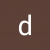# Water in Space Engineers (yes I get it, it's hard to implement, but hear me out)

C.DEF shared this feedback ago
Submitted

So we know that simulating water in a game where limits are customizable is very difficult and might require rewriting VRage, but there might be workarounds.

Water, if you didn't know already, clumps up into a sphere when in zero gravity. What if we map points in space that are attracted to each other but only at a certain distance (lets say 0.5 Meters) and that each point has a radius compared to how many kg are there using this equation:

```M=Mass | De=Density | V=Volume | Di=Diameter | R=Radius

{M÷De=3√((V*3)÷4π)=Di/2=R}

Ex.

{1000Kg ÷ 997Kg/m3 = 1.00300902708Kg}

{1.00300902708 * 3 = 3.00902708124}

{3.00902708124÷4π = 0.23945076693}

{3√0.23945076693 = 1.46801120649}

{1.46801120649÷2=0.73400560324M}```

When they do get close enough to merge, they would add masses and recalculate the new radius. In order to display the water, a sphere would be put into place and scaled based on the radius (the sphere would initially be 0.5 meters in radius).

If water were to enter an artificial gravity field, it would fall down in 1 direction as a single mass as there would be no air resistance. As soon as it touches a voxel or grid, then there would need to be many more physics calculations to render the deformation of the water (such as ray-tracing on the edge of the sphere to detect if it hit a voxel/grid or not, and then the water would need to split into many new points, each with a velocity and direction).

The complications of entering a planet would be strange, especially for planets with atmospheres. Questions such as "Would the water evaporate as it fell through the atmosphere?" or "Would the water need to split up into many pieces due to air resistance?" Water would need to stretch its shape and lose mass/split as it falls, and any higher speeds from mods would probably make the water crash through the planet. The same ray-tracing and splitting would be done with voxels and grids on planets.

The hardest thing to implement would be deformation from entire ships, as the water would need to compress and move mass out of the way, which would be so incredibly laggy that you'd be better off crashing into ice.

These are just purely hypothetical solutions to the problem and would be extremely challenging to implement.3

how would you calculate a ship ramming into it? splashing?(=heavy simulation of lots of small particles with collision detection) would it stick to surfaces and whats the use in space?

most people want water as in seas and floating ships on it and not accurate simulation . but there is the problem with voxels and how to detect if a ship floats.

your approach needs an algorithm that takes the lowest point in the region and has to calculate all the points of the voxel mesh and return a volume between them. for small amounts like a pool full itmight be doable in the frame time(when editing voxels and adjusting the weight of a ship). but seas? oceans?

voxels: what do you do when you drill a hole near the water? is it just always filled with water until a certain level or would you calculate each droplet to run down into the hole(then you have to re calculate the whole sea)? if you extend the sea far out with drills. is it still the same level everywhere?

so it can be a fixed sphere inside a planet for only oceans but if you drill anywhere on the planet is the hole from a certain point just filled with water?. but seas anywhere above it would have to be ice..

ship floating: a complex algorithm would have to be in place. which is doable like how much does the ship weight/ how much space is being used by side nearest to the center of gravity of the ship. aka the blocks in there. (if it slightly tilted around 3 axis it gets a complicated calculation) then you have to check if those blocks are all watertight (more complicated then airtight because of up to 3 open sides and a much bigger structure.).

what do you do with the water level inside the ship?you could not render the water level within the (watertight space which requires realtime calculations) like how do you implement sinking/water getting in. what about trapped air inside.

so many computational things that would bring the game to a stop. and every reduction in use make it seem bad.. not forgetting the players who game on lower end pcs1

In the Dual Universes, water was somehow made together with snow on the planet (voxel world). Moreover, the system requirements for the game are almost the same. Or laziness or a strong difference in engines.